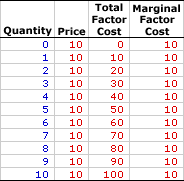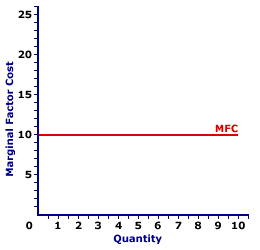Thursday  May 26, 2022
 AmosWEB means Economics with a Touch of Whimsy!EDUCATION: An increase in the knowledge or skills processed by people. Education is generally view from a "formal" perspective, in which "students" sit in classrooms attuned to enthralling lectures from teachers before they are forced to reveal their education through exams. Education, however, can be much less formal, acquired on the job or through the real word experiences. An important economic aspect of is how it enhances the productivity of labor by increasing human capital. Education, in fact, has been one of the prime sources of economic growth and improved living standards.MARGINAL FACTOR COST, PERFECT COMPETITION:

The change in total factor cost resulting from a change in the quantity of factor input employed by a perfectly competitive firm. Marginal factor cost, abbreviated MFC, indicates how total factor cost changes with the employment of one more input. It is found by dividing the change in total factor cost by the change in the quantity of input used. Marginal factor cost is compared with marginal revenue product to identify the profit-maximizing quantity of input to hire.
Marginal factor cost is the extra cost incurred when a perfectly competitive firm buys one more unit of an input. It plays THE key role in the study of factor markets and the profit maximizing decision of a perfectly competitive firm relative to marginal revenue product. A perfectly competitive firm maximizes profit by equating marginal revenue product, the extra revenue generated from using an input, with marginal factor cost, the extra cost incurred by using the input. If these two marginals are not equal, then profit can be increased by hiring more or less input.

The relation between marginal factor cost and quantity of input used depends on market structure. For a perfectly competitive firm, marginal factor cost is equal to factor price and average factor cost, all of which are constant. For a monopsony, monopsonistically competitive, or oligopsony firm, marginal factor cost is greater than average factor cost and factor price, all of which increase with larger quantities of input. The constant or increasing nature of marginal factor cost is a prime indication of the market control of a firm.

Whichever market structure is involved, marginal factor cost is calculated as the change in total factor cost divided by the change in the quantity of the factor purchased, as illustrated by this equation:

 marginal factor cost = change in total factor costchange in factor quantity
If the firm is hiring the factor in a perfectly competitive factor market, then the factor price is fixed or constant and so too is marginal factor cost. If the firm is hiring the factor in an imperfectly competitive factor market, best illustrated by monopsony, then the factor price increases with larger factor quantities and so too does marginal factor cost.

Perfect competition is a market structure with a large number of small participants (buyers and sellers). The good exchanged in the market is identical, regardless of who sells or who buys. Participants have perfect knowledge and perfect mobility into and out of the market. These conditions mean perfectly competitive buyers are price takers, they have no market control and must pay the going market price for all input bought.

Marginal Factor Cost,
Perfect CompetitionThe table to the right summarizes the marginal factor cost incurred by a hypothetical buyer, Maggie's Macrame Shoppe, for hiring store clerks in a perfectly competitive labor market. Maggie's Macrame Shoppe is one of thousands of small retail stores in the greater Shady Valley metropolitan area that hires labor with identical skills. As such, Maggie pays the going wage for labor.

The first column in the table is the quantity of workers hired, ranging from 0 to 10 workers. The second column is the price Maggie pays for hiring her workers, which is constant at \$10 per worker. The third column is the total factor cost Maggie incurs for hiring various numbers of workers. If Maggie hires only one worker, then she pays only \$10. If she hires five workers, she pays \$50. In each case, total factor cost in column three is calculated as the quantity in the first column multiplied by the price in the second column.

The fourth column then presents the marginal factor cost incurred by Maggie at each level of employment. These numbers are found by dividing the change in total factor cost in the third column by the change in factor quantity in the first column. For example, when Maggie increases employment from 4 workers to 5 workers, her total factor cost increases from \$40 to \$50, an increase of \$10. As such, the marginal factor cost of hiring the fifth worker is \$10 (= \$10/1). Each value in the fourth column is calculated in the same way.

The two key observations about marginal factor cost are:

• First, marginal factor cost is constant. Each level of employment results in the same \$10 marginal factor cost.

• Second, marginal factor cost is equal to price (which is also average factor cost) at every level of employment. This results because Maggie is operating in a perfectly competitive labor market.
Marginal Factor Cost Curve,
Perfect CompetitionMarginal factor cost is commonly represented by a marginal factor cost curve, such as the one labeled MFC and displayed in the exhibit to the right. This particular marginal factor cost curve is that for labor hired by Maggie's Macrame Shoppe.

The vertical axis measures marginal factor cost and the horizontal axis measures the quantity of input (workers). Although quantity on this particular graph stops at 10 workers, the nature of perfect competition indicates it could easily go higher.

First and foremost, the marginal factor cost curve is horizontal at the going factor price of \$10. This indicates that if Maggie hires the first worker, then her total factor cost increases by \$10. Alternatively, if she hires the tenth worker, then her total factor cost increases by \$10. Should she hire a hundredth worker, then she might move well beyond the graph, but her total factor cost increases by \$10.

The "curve" is actually a "straight line" because Maggie is a price taker in the labor market. She pays \$10 for each worker whether she hires 1 worker or 10 workers or 100 workers. Her extra factor cost of hiring an extra worker is always \$10. The constant price is what makes Maggie's marginal factor cost curve a straight line, and which indicates that Maggie has no market control.

 <= MARGINAL FACTOR COST, MONOPSONY MARGINAL PHYSICAL PRODUCT =>Recommended Citation:

MARGINAL FACTOR COST, PERFECT COMPETITION, AmosWEB Encyclonomic WEB*pedia, http://www.AmosWEB.com, AmosWEB LLC, 2000-2022. [Accessed: May 26, 2022].

Check Out These Related Terms...

Or For A Little Background...

And For Further Study...
Search Again?YELLOW CHIPPEROON[What's This?] Today, you are likely to spend a great deal of time looking for a downtown retail store looking to buy either a flower arrangement in a coffee cup for your father or a how-to book on meeting people. Be on the lookout for malfunctioning pocket calculators.Your Complete ScopeA half gallon milk jug holds about \$50 in pennies."Try not to become a man of success, but rather try to become a man of value. "-- Albert EinsteinARAverage Revenue, AutoregressiveA PEDestrian's Guide Xtra CreditTell us what you think about AmosWEB. Like what you see? Have suggestions for improvements? Let us know. Click the User Feedback link.| | | | | | | | | | |
| | | |

Thanks for visiting AmosWEB